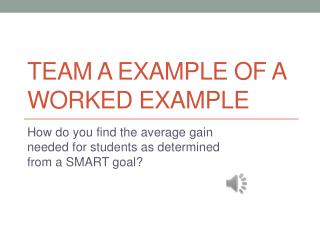DownloadDownload PresentationTeam A Example of a Worked Example

# Team A Example of a Worked Example

Download Presentation## Team A Example of a Worked Example

- - - - - - - - - - - - - - - - - - - - - - - - - - - E N D - - - - - - - - - - - - - - - - - - - - - - - - - - -
##### Presentation Transcript

1. Team A Example of a Worked Example How do you find the average gain needed for students as determined from a SMART goal?

2. How To Find The Gains For SMART Goals SMART S=Specific M=Measureable A= Aligned R= Realistic T=Timely

3. SMART Goal: Whole Class • Kelsey’s 4th grade virtual class will make an average 10% proficiency gains in Reading according to Standard Test Scores by May 2013.

4. Kelsey Elementary School/4th Grade • Kelsey’s 4th grade virtual class will make an average 10% proficiency gains in Reading according to Standard Test Scores by May 2013.

5. Finding Average Score • 78+73+67+43+91+47+81+58+79+94+79+100+91+48+54+93+88+95+67+89= 1515 (Virtual Organization Portal, 2013)

6. Finding Average Score • 1515÷20= • 75.75 • 75.75 is the average Reading score for this class. Our SMART goal is to make a 10% increase from this number.

7. What score is 10% Higher than 75.75? • To find a percent of a number you divide your percent by 100 and multiply the decimal to the number • 10/100=.10 • 10% of 75.75 is • .10 X 75.75= • 7.575

8. New Score to Strive For • Average score= 75.75 • Add 10%= 7.575 • 75.75+7.575= 83.325 or the next whole number, 84

9. SMART Goal: Whole Class • Kelsey’s 4th grade virtual class will make an average 10% proficiency gains in Reading according to Standard Test Scores by May 2013.

10. SMART Goal: Individual Student • Alex will raise his proficiency in Reading by 10% according to Standard Test Scores by May 2013.

11. Alex’s Score • What is 10% of Alex’s current Reading Score? • Current score= 78 • 78X.10=7.8 • Why do we multiply 78 by .10? • 10%=1/100=.10 • What do you do with 7.8 now?

12. Alex’s Goal Score for Reading • Current Score=78 • Add 10%= 7.8 • Goal= 78+7.8= SMART Goal is 85.8

13. References • Virtual Organization Portal. (2013). Kelsey Elementary School. Retrieved from https://ecampus.phoenix.edu/secure/aapd/cist/vop/Education/Elem/ElemHome.asp